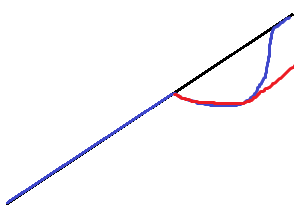# Unit Root: Simple Definition, Unit Root Tests

Time Series > Unit Root

## What is “Unit Root”?

A unit root (also called a unit root process or a difference stationary process) is a stochastic trend in a time series, sometimes called a “random walk with drift”; If a time series has a unit root, it shows a systematic pattern that is unpredictable.A possible unit root. The red line shows the drop in output and path of recovery if the time series has a unit root. Blue shows the recovery if there is no unit root and the series is trend-stationary.

## The Mathematics Behind Unit Roots

The reason why it’s called a unit root is because of the mathematics behind the process. At a basic level, a process can be written as a series of monomials (expressions with a single term). Each monomial corresponds to a root. If one of these roots is equal to 1, then that’s a unit root.

The math behind unit roots is beyond the scope of this site (although if you’re really interested you can read about it in this pdf). All you really need to know if you’re analyzing time series is that the existence of unit roots can cause your analysis to have serious issues like:

• Spurious regressions: you could get high r-squared values even if the data is uncorrelated.
• Errant behavior due to assumptions for analysis not being valid. For example, t-ratios will not follow a t-distribution.

## What is a Unit Root Test?

Unit root tests are tests for stationarity in a time series. A time series has stationarity if a shift in time doesn’t cause a change in the shape of the distribution; unit roots are one cause for non-stationarity.

These tests are known for having low statistical power. Many tests exist, in part, because none stand out as having the most power. Tests include:

• The Dickey Fuller Test (sometimes called a Dickey Pantula test), which is based on linear regression. Serial correlation can be an issue, in which case the Augmented Dickey-Fuller (ADF) test can be used. The ADF handles bigger, more complex models. It does have the downside of a fairly high Type I error rate.
• The Elliott–Rothenberg–Stock Test, which has two subtypes:
• The P-test takes the error term’s serial correlation into account,
• The DF-GLS test can be applied to detrended data without intercept.
• The Schmidt–Phillips Test includes the coefficients of the deterministic variables in the null and alternate hypotheses. Subtypes are the rho-test and the tau-test.
• The Phillips–Perron (PP) Test is a modification of the Dickey Fuller test, and corrects for autocorrelation and heteroscedasticity in the errors.
• The Zivot-Andrews test allows a break at an unknown point in the intercept or linear trend.

## References

Kotz, S.; et al., eds. (2006), Encyclopedia of Statistical Sciences, Wiley.
Everitt, B. S.; Skrondal, A. (2010), The Cambridge Dictionary of Statistics, Cambridge University Press.
Vogt, W.P. (2005). Dictionary of Statistics & Methodology: A Nontechnical Guide for the Social Sciences. SAGE.

CITE THIS AS:
Stephanie Glen. "Unit Root: Simple Definition, Unit Root Tests" From StatisticsHowTo.com: Elementary Statistics for the rest of us! https://www.statisticshowto.com/unit-root/
---------------------------------------------------------------------------Need help with a homework or test question? With Chegg Study, you can get step-by-step solutions to your questions from an expert in the field. Your first 30 minutes with a Chegg tutor is free!